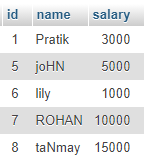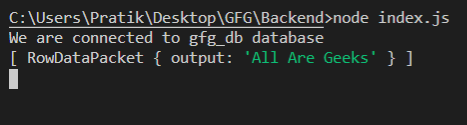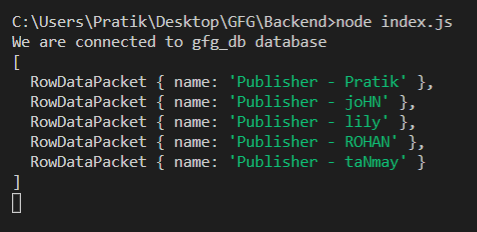GFG App
Open AppBrowser
Continue

# Node.js MySQL INSERT() Function

INSERT() function is a built-in function in MySQL that is used to insert a string into another string at some position after deleting some characters from it.

Syntax:

`INSERT(string_1, position, number_of_characters_to_delete, string_2)`

Parameters: It takes four parameters as follows:

• string_1: It is the given main string passed as a parameter.
• position: The string_2 will insert at this position.
• number_of_characters_to_delete: It is the number of characters to be deleted from string_1 at a given position.
• string_2: It is the given new string for insertion.

Return Value: It returns a new string after deleting characters from string_1 then inserting string_2.

Module Installation: Install the mysql module using the following command:

`npm install mysql`

Database: Our SQL publishers table preview with sample data is shown below:Example 1:

## index.js

 `const mysql = require(``"mysql"``); ` ` `  `let db_con  = mysql.createConnection({ ` `    ``host: ``"localhost"``, ` `    ``user: ``"root"``, ` `    ``password: ``''``, ` `    ``database: ``'gfg_db'` `}); ` ` `  `db_con.connect((err) => { ` `    ``if` `(err) { ` `      ``console.log(``"Database Connection Failed !!!"``, err); ` `      ``return``; ` `    ``} ` ` `  `    ``console.log(``"We are connected to gfg_db database"``); ` ` `  `    ``// Here is the query ` `    ``let query =  ` `"SELECT INSERT('GeeksforGeeks', 1, 8, 'All Are ') AS output"``; ` ` `  `    ``db_con.query(query, (err, rows) => { ` `        ``if``(err) ``throw` `err; ` ` `  `        ``console.log(rows); ` `    ``}); ` `});`

Run the index.js file using the following command:

`node index.js`

Output: Here, the first 8 characters are deleted from ‘GeeksforGeeks’ from position 1 – ‘Geeks’. Then string_2 is inserted – ‘All Are Geeks’Example 2:

## index.js

 `const mysql = require(``"mysql"``); ` ` `  `let db_con  = mysql.createConnection({ ` `    ``host: ``"localhost"``, ` `    ``user: ``"root"``, ` `    ``password: ``''``, ` `    ``database: ``'gfg_db'` `}); ` ` `  `db_con.connect((err) => { ` `    ``if` `(err) { ` `      ``console.log(``"Database Connection Failed !!!"``, err); ` `      ``return``; ` `    ``} ` ` `  `    ``console.log(``"We are connected to gfg_db database"``); ` ` `  `    ``// Here is the query ` `    ``let query =  ` `"SELECT INSERT(name, 1, 0, 'Publisher - ') AS name FROM publishers"``; ` ` `  `    ``db_con.query(query, (err, rows) => { ` `        ``if``(err) ``throw` `err; ` ` `  `        ``console.log(rows); ` `    ``}); ` `});`

Run the index.js file using the following command:

`node index.js`

Output:My Personal Notes arrow_drop_up